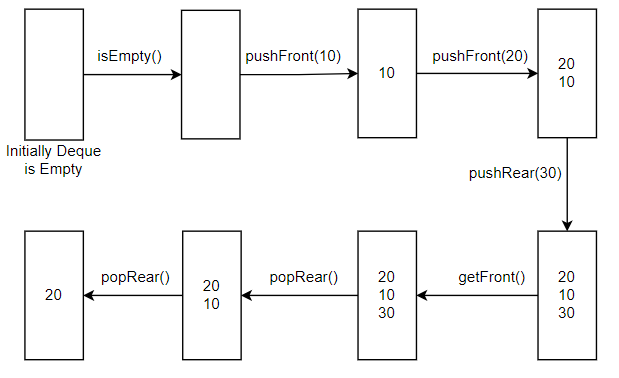Problem title
Difficulty
Avg time to solve

Implement Deque
Easy
15 mins
Valid Stack Permutation
Moderate
45 mins
Save The World
Easy
15 mins
Partition
Easy
10 mins
Shape and Overriding
Easy
--
Minimal Tree
Easy
15 mins
Bellman Ford
Moderate
30 mins
Maximum Time
Easy
15 mins
Construct BST from Preorder Traversal
Moderate
--
Loudspeakers
Moderate
20 mins11

# Implement Deque

Difficulty: EASY
Avg. time to solve
15 min
Success Rate
85%

Problem Statement

#### Design a data structure to implement deque of size ‘N’. It should support the following operations:

``````pushFront(X): Inserts an element X in the front of the deque. Returns true if the element is inserted, otherwise false.

pushRear(X): Inserts an element X in the back of the deque. Returns true if the element is inserted, otherwise false.

popFront(): Pops an element from the front of the deque. Returns -1 if the deque is empty, otherwise returns the popped element.

popRear(): Pops an element from the back of the deque. Returns -1 if the deque is empty, otherwise returns the popped element.

getFront(): Returns the first element of the deque. If the deque is empty, it returns -1.

getRear(): Returns the last element of the deque. If the deque is empty, it returns -1.

isEmpty(): Returns true if the deque is empty, otherwise false.

isFull(): Returns true if the deque is full, otherwise false.
``````

#### Following types of queries denote these operations:

``````Type 1: for pushFront(X) operation.
Type 2: for pushRear(X) operation.
Type 3: for popFront() operation.
Type 4: for popRear() operation.
Type 5: for getFront() operation.
Type 6: for getRear() operation.
Type 7: for isEmpty() operation.
Type 8: for isFull() operation.
``````
##### Input format:
``````The first line of input contains two space-separated integers ‘N’ and ‘Q’ denoting the size of the deque and the number of queries to be performed, respectively.

The next ‘Q’ lines specify the type of operation/query to be performed on the data structure.

Each query contains an integer ‘P’ denoting the type of query.

For the query of type 1 and 2, the integer ‘P’ is followed by a single integer ‘X’ denoting the element on which operation is to be performed.

For the queries of type 3 to 8, a single integer ‘P’ is given, denoting the type of query.
``````
##### Output format:
``````For each query, print the output returned after performing the corresponding operation on the data structure.
``````
##### Note:
``````You do not need to print anything, it has already been taken care of. Just implement the given functions.
``````
##### Constraints:
``````1 <= N <= 1000
1 <= Q <= 10^5
1 <= P <= 8
1 <= X <= 10^5

Time Limit: 1 sec

Where ‘N’ represents the size of the deque, ‘Q’ represents the number of queries, ‘P’ represents the type of operation and ‘X’ represents the element.
``````
##### Sample Input 1:
``````5 7
7
1 10
1 20
2 30
5
4
4
``````
##### Sample Output 1:
``````True
True
True
True
20
30
10
``````
##### Explanation 1:
``````For the given input, we have the number of queries, Q = 7.
Operations performed on the deque are as follows:

isEmpty(): Deque is initially empty. So, this returns true.
pushFront(10): Insert the element ‘10’ in the front of the deque. This returns true.
pushFront(20): Insert the element ‘20’ in the front of the deque. This returns true.
pushRear(30): Insert the element ‘30’ in the back of the deque. This returns true.
getFront(): Returns the front element of the deque i.e. 20
popRear(): Pop an element from the back of the deque. This returns 30.
popRear(): Pop an element from the back of the deque. This returns 10.

The following image shows the snapshots of the deque after each operation:
``````##### Sample Input 2:
``````2 5
1 15
2 25
1 20
8
6
``````
##### Sample Output 2:
``````True
True
False
True
25
``````Console